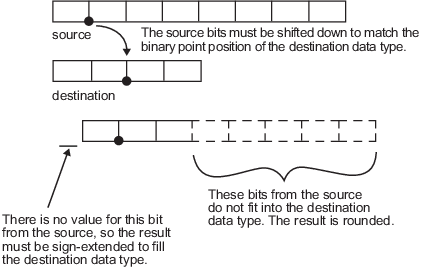## 算术运算

### 模算术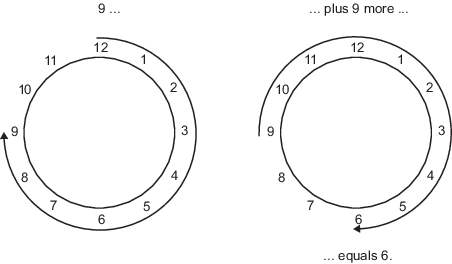### 2 的补码

2 的补码是解释二进制数字的一种方式。在 2 的补码中，正数始终以 0 开头，负数始终以 1 开头。如果 2 的补码数的前导位为 0，则通过计算该数的标准二进制值来获得该值。如果 2 的补码数的前导位是 1，则通过假设最左侧位是负数然后计算该数的二进制值来获得该值。例如，

`$\begin{array}{l}01=\left(0+{2}^{0}\right)=1\\ 11=\left(\left(-{2}^{1}\right)+\left({2}^{0}\right)\right)=\left(-2+1\right)=-1\end{array}$`

1. 获得 1 的补码，即“翻转位”。

2. 按二进制数学计算方式加 2^(-FL)，其中 FL 是小数长度。

3. 丢弃超出原始字长的任何位。

`$11010\to 00101$`

`$\begin{array}{l}\underset{¯}{\begin{array}{r}00101\\ +1\end{array}}\\ \begin{array}{cc}00110& \left(6\right)\end{array}\end{array}$`

### 加减法

`$\begin{array}{cc}\begin{array}{r}\underset{¯}{\begin{array}{c}010010.1\\ +0110.110\end{array}}\\ 011001.010\end{array}& \begin{array}{l}\left(18.5\right)\hfill \\ \left(6.75\right)\hfill \\ \left(25.25\right)\hfill \end{array}\end{array}$`

`$\begin{array}{cc}\underset{¯}{\begin{array}{c}010010.100\\ -0110.110\end{array}}& \begin{array}{c}\left(18.5\right)\\ \left(6.75\right)\end{array}\end{array}$`

### 乘法

2 的补码定点数的乘法与普通小数乘法几乎完全一样，只是中间结果必须经过符号扩展，以便在它们相加之前将其左侧对齐。

`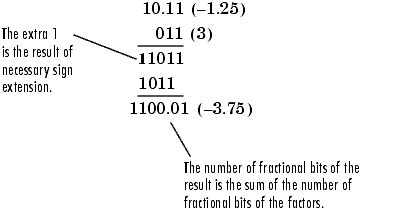`

#### 乘法数据类型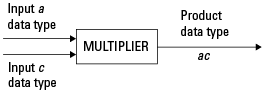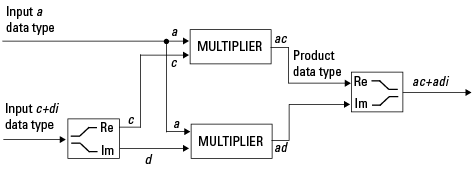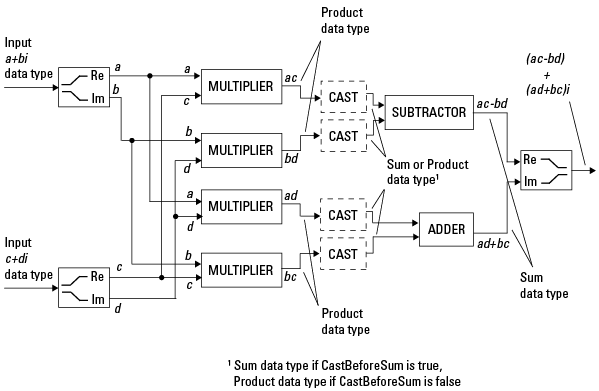`fimath` 对象的 `CastBeforeSum` 属性为 `true` 时，在上图中的乘数后将转换为加和数据类型。在 C 代码中，对于减法器，这相当于

```acc=ac; acc-=bd;```

```acc=ad; acc+=bc;```

#### 与 fimath 相乘

```F = fimath('ProductMode','FullPrecision',... 'SumMode','FullPrecision'); T1 = numerictype('WordLength',24,'FractionLength',20); T2 = numerictype('WordLength',16,'FractionLength',10);```

```P = fipref; P.FimathDisplay = 'none'; x = fi(5,T1,F)```
```x = 5 DataTypeMode: Fixed-point: binary point scaling Signedness: Signed WordLength: 24 FractionLength: 20```
`y = fi(10,T2,F)`
```y = 10 DataTypeMode: Fixed-point: binary point scaling Signedness: Signed WordLength: 16 FractionLength: 10```
`z = x*y`
```z = 50 DataTypeMode: Fixed-point: binary point scaling Signedness: Signed WordLength: 40 FractionLength: 30```

`x = fi(5,T1,F)`
```x = 5 DataTypeMode: Fixed-point: binary point scaling Signedness: Signed WordLength: 24 FractionLength: 20```
`y = fi(10+2i,T2,F)`
```y = 10.0000 + 2.0000i DataTypeMode: Fixed-point: binary point scaling Signedness: Signed WordLength: 16 FractionLength: 10```
`z = x*y`
```z = 50.0000 +10.0000i DataTypeMode: Fixed-point: binary point scaling Signedness: Signed WordLength: 40 FractionLength: 30```

`x = fi(5+6i,T1,F)`
```x = 5.0000 + 6.0000i DataTypeMode: Fixed-point: binary point scaling Signedness: Signed WordLength: 24 FractionLength: 20```
`y = fi(10+2i,T2,F)`
```y = 10.0000 + 2.0000i DataTypeMode: Fixed-point: binary point scaling Signedness: Signed WordLength: 16 FractionLength: 10```
`z = x*y`
```z = 38.0000 +70.0000i DataTypeMode: Fixed-point: binary point scaling Signedness: Signed WordLength: 41 FractionLength: 30```

### 转换

`fimath` 对象允许您使用 `SumMode``ProductMode` 属性指定中间求和与乘积的数据类型和定标。当您设置 `SumMode``ProductMode` 属性时，务必考虑每种转换会产生的影响。根据您选择的数据类型，可能会发生溢出和/或舍入。以下两个示例说明可能发生溢出和舍入的情况。

#### 从较短的数据类型转换为较长的数据类型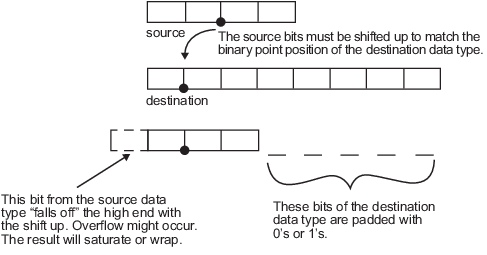• 如果没有发生溢出，空位将用 0 填充。

• 如果发生绕回，空位将用 0 填充。

• 如果发生饱和，

• 正数的空位用 1 填充。

• 负数的空位用 0 填充。

#### 从较长的数据类型转换为较短的数据类型